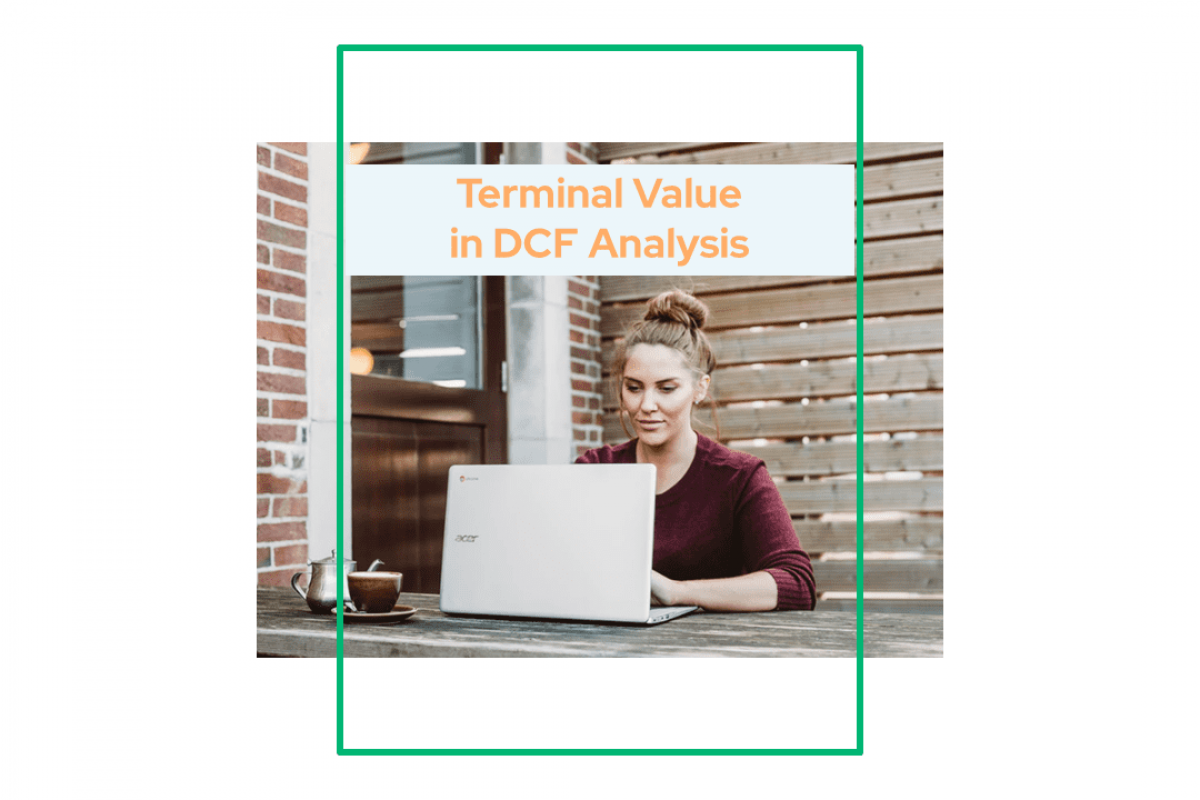# Terminal Value in DCF AnalysisKeyskillset
15
August
2019### What is Terminal Value (TV)?

In finance, the terminal value in DCF Analysis(also “continuing value” or “horizon value”) of a security is the present value at a future point in time of all future cash flows. We expect this to happen at a stable growth rate forever. We use it mostly in a multi-stage discounted cash flow analysis. Besides, it allows for the limitation of cash flow projections to a several-year period; see the Forecast period (finance).

Forecasting results beyond such a period is impractical. In other words, it exposes such projections to a variety of risks limiting their validity. Primarily, the great uncertainty involved in predicting industry and macroeconomic conditions beyond a few years.

Thus, the terminal value in DCF Analysis allows for the inclusion of the value of future cash flows. Those cash flows occur beyond a several-year projection period. At the same time, the terminal value satisfactorily mitigates many of the problems of valuing such cash flows. We calculate the terminal value in accordance with a stream of projected future free cash flows in discounted cash flow analysis. For example, for valuation purposes, the company can use two methodologies to calculate the Terminal Value.

### 1) Perpetuity Growth Method

Perpetual Growth Method is also known as the Gordon Growth Perpetual Model. This is the most preferred method. We assume that the growth of the company will continue. Also, the return on capital will be more than the cost of capital.

Here’s the formula for Terminal Value calculated with the perpetuity growth method:

(FCF * (1 + g)) / (WACC – g), where:

FCF = Free cash flow for the last forecast period

g = Terminal growth rate

WACC = discount rate (weighted average cost of capital)

### 2) Exit Multiple Method

We use the Exit Multiple Method with assumptions that market multiple bases to value a business. The terminal multiple can be the enterprises’ value/ EBITDA or enterprise value/EBIT. For instance, these are the usual multiples used in financial valuation. The projected statistics are the relevant statistics that we projected in the previous year.

Here’s the formula for Terminal Value calculated with the exit multiple method:

Terminal Value = Last Twelve months Terminal Multiple * Projected Statistic

### How to calculate the Terminal Value in DCF Analysis?

Terminal value (TV) is the value of a business or project beyond the forecast period when future cash flows can be estimated. Terminal value assumes the business will grow at a set growth forever after the forecast period.

Here’s the formula to calculate terminal value:

Terminal Value = (Unlevered FCF for the last projected year* ( 1 + Growth Rate ))/ ( WACC – Growth Rate)

You can find the calculation of Terminal Value for our sample model in the screenshot below.

### Conclusion

• Terminal value (TV) determines a company’s value into perpetuity beyond a set forecast period—usually five years.
• Analysts use the discounted cash flow model (DCF) to calculate the total value of a business. Eventually, DCF has two major components—the forecast period and terminal value.
• We can calculate the terminal value by using two methodologies—perpetual growth (Gordon Growth Model) and exit multiple.
• The perpetual growth method assumes that a business will continue to generate cash flows at a constant rate forever. Meanwhile, the Exit Multiple Method assumes that a business will be sold for a multiple of some market metrics.